Anzeige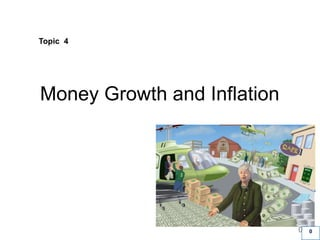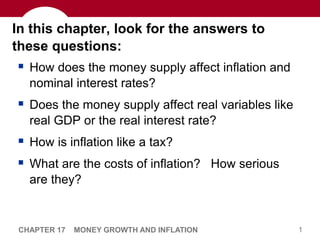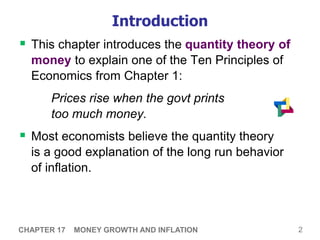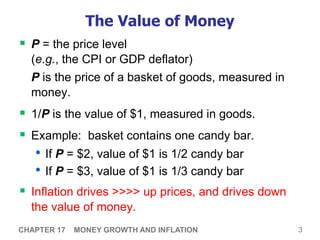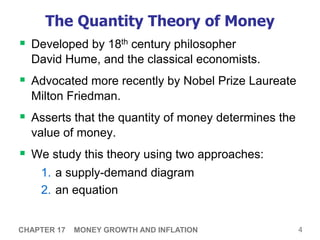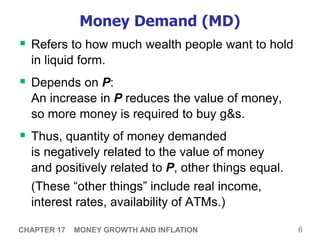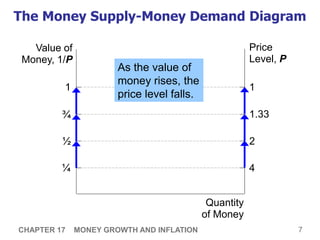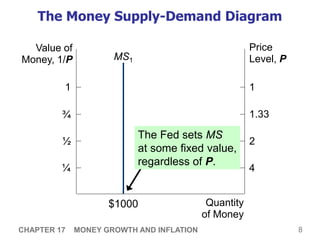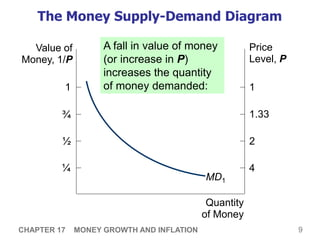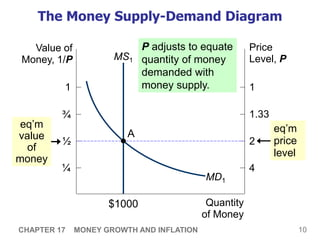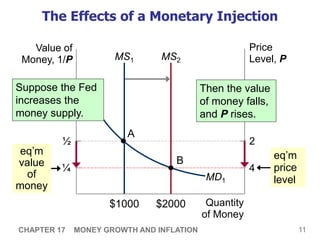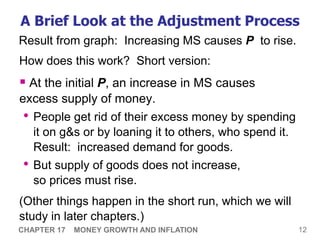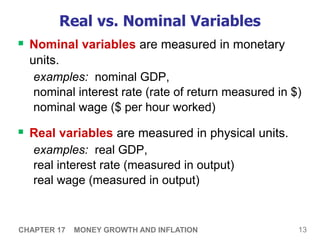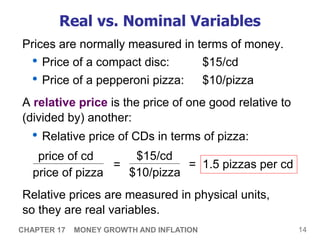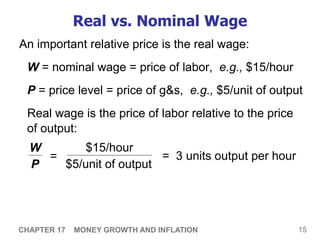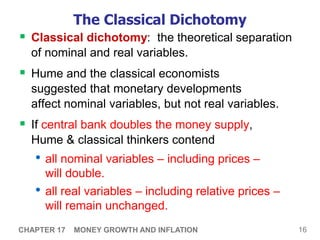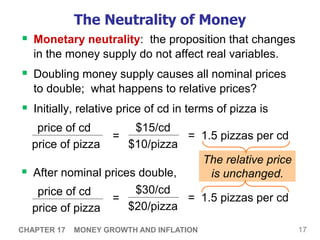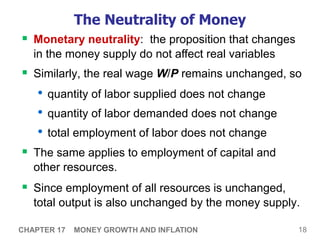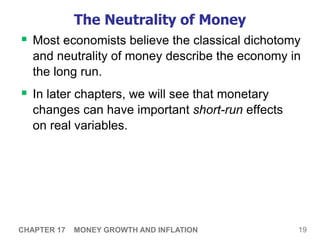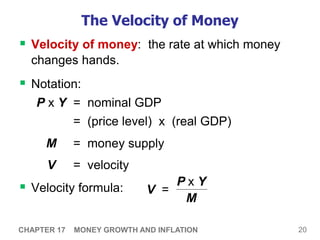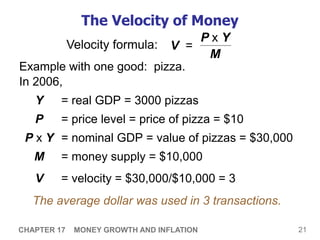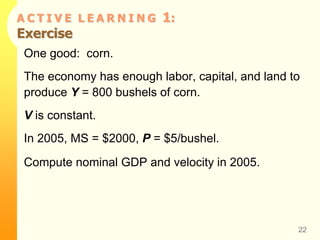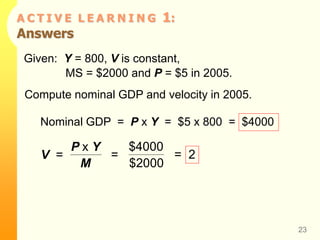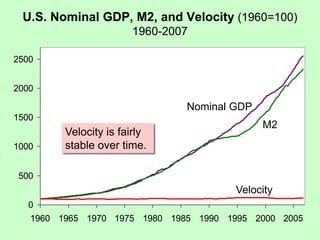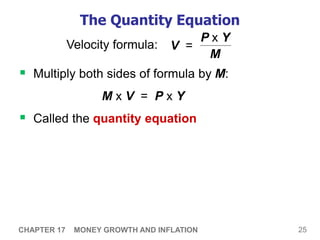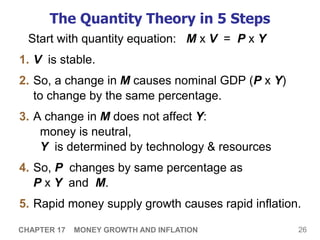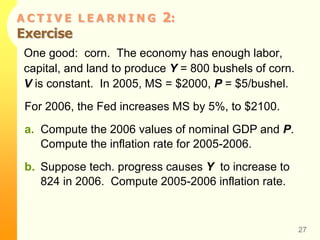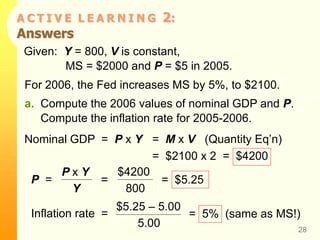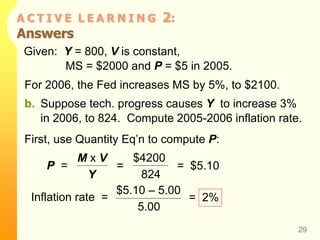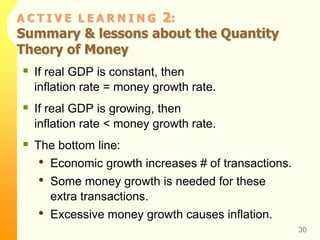1 von 31
Anzeige

### Macro L5.ppt

1. 0 PowerPoint Slides prepared by: Andreea CHIRITESCU Eastern Illinois University 30 Money Growth and Inflation 0 Topic 4
2. 1 CHAPTER 17 MONEY GROWTH AND INFLATION In this chapter, look for the answers to these questions:  How does the money supply affect inflation and nominal interest rates?  Does the money supply affect real variables like real GDP or the real interest rate?  How is inflation like a tax?  What are the costs of inflation? How serious are they?
3. 2 CHAPTER 17 MONEY GROWTH AND INFLATION Introduction  This chapter introduces the quantity theory of money to explain one of the Ten Principles of Economics from Chapter 1: Prices rise when the govt prints too much money.  Most economists believe the quantity theory is a good explanation of the long run behavior of inflation.
4. 3 CHAPTER 17 MONEY GROWTH AND INFLATION The Value of Money  P = the price level (e.g., the CPI or GDP deflator) P is the price of a basket of goods, measured in money.  1/P is the value of \$1, measured in goods.  Example: basket contains one candy bar. • If P = \$2, value of \$1 is 1/2 candy bar • If P = \$3, value of \$1 is 1/3 candy bar  Inflation drives >>>> up prices, and drives down the value of money.
5. 4 CHAPTER 17 MONEY GROWTH AND INFLATION The Quantity Theory of Money  Developed by 18th century philosopher David Hume, and the classical economists.  Advocated more recently by Nobel Prize Laureate Milton Friedman.  Asserts that the quantity of money determines the value of money.  We study this theory using two approaches: 1. a supply-demand diagram 2. an equation
6. 5 CHAPTER 17 MONEY GROWTH AND INFLATION Money Supply (MS)  In real world, determined by Federal Reserve, the banking system, consumers.  In this model, we assume the Fed precisely controls MS and sets it at some fixed amount.
7. 6 CHAPTER 17 MONEY GROWTH AND INFLATION Money Demand (MD)  Refers to how much wealth people want to hold in liquid form.  Depends on P: An increase in P reduces the value of money, so more money is required to buy g&s.  Thus, quantity of money demanded is negatively related to the value of money and positively related to P, other things equal. (These “other things” include real income, interest rates, availability of ATMs.)
8. 7 CHAPTER 17 MONEY GROWTH AND INFLATION The Money Supply-Money Demand Diagram Value of Money, 1/P Price Level, P Quantity of Money 1 1 ¾ 1.33 ½ 2 ¼ 4 As the value of money rises, the price level falls.
9. 8 CHAPTER 17 MONEY GROWTH AND INFLATION The Money Supply-Demand Diagram Value of Money, 1/P Price Level, P Quantity of Money 1 ¾ ½ ¼ 1 1.33 2 4 MS1 \$1000 The Fed sets MS at some fixed value, regardless of P.
10. 9 CHAPTER 17 MONEY GROWTH AND INFLATION The Money Supply-Demand Diagram Value of Money, 1/P Price Level, P Quantity of Money 1 ¾ ½ ¼ 1 1.33 2 4 MD1 A fall in value of money (or increase in P) increases the quantity of money demanded:
11. 10 CHAPTER 17 MONEY GROWTH AND INFLATION MS1 \$1000 Value of Money, 1/P Price Level, P Quantity of Money 1 ¾ ½ ¼ 1 1.33 2 4 The Money Supply-Demand Diagram MD1 P adjusts to equate quantity of money demanded with money supply. eq’m price level eq’m value of money A
12. 11 CHAPTER 17 MONEY GROWTH AND INFLATION MS1 \$1000 The Effects of a Monetary Injection Value of Money, 1/P Price Level, P Quantity of Money 1 ¾ ½ ¼ 1 1.33 2 4 MD1 eq’m price level eq’m value of money A MS2 \$2000 B Then the value of money falls, and P rises. Suppose the Fed increases the money supply.
13. 12 CHAPTER 17 MONEY GROWTH AND INFLATION A Brief Look at the Adjustment Process How does this work? Short version:  At the initial P, an increase in MS causes excess supply of money. • People get rid of their excess money by spending it on g&s or by loaning it to others, who spend it. Result: increased demand for goods. • But supply of goods does not increase, so prices must rise. (Other things happen in the short run, which we will study in later chapters.) Result from graph: Increasing MS causes P to rise.
14. 13 CHAPTER 17 MONEY GROWTH AND INFLATION Real vs. Nominal Variables  Nominal variables are measured in monetary units. examples: nominal GDP, nominal interest rate (rate of return measured in \$) nominal wage (\$ per hour worked)  Real variables are measured in physical units. examples: real GDP, real interest rate (measured in output) real wage (measured in output)
15. 14 CHAPTER 17 MONEY GROWTH AND INFLATION Real vs. Nominal Variables Prices are normally measured in terms of money. • Price of a compact disc: \$15/cd • Price of a pepperoni pizza: \$10/pizza A relative price is the price of one good relative to (divided by) another: • Relative price of CDs in terms of pizza: price of cd price of pizza \$15/cd \$10/pizza = Relative prices are measured in physical units, so they are real variables. = 1.5 pizzas per cd
16. 15 CHAPTER 17 MONEY GROWTH AND INFLATION Real vs. Nominal Wage An important relative price is the real wage: W = nominal wage = price of labor, e.g., \$15/hour P = price level = price of g&s, e.g., \$5/unit of output Real wage is the price of labor relative to the price of output: W P = 3 units output per hour \$15/hour \$5/unit of output =
17. 16 CHAPTER 17 MONEY GROWTH AND INFLATION The Classical Dichotomy  Classical dichotomy: the theoretical separation of nominal and real variables.  Hume and the classical economists suggested that monetary developments affect nominal variables, but not real variables.  If central bank doubles the money supply, Hume & classical thinkers contend • all nominal variables – including prices – will double. • all real variables – including relative prices – will remain unchanged.
18. 17 CHAPTER 17 MONEY GROWTH AND INFLATION The Neutrality of Money  Monetary neutrality: the proposition that changes in the money supply do not affect real variables.  Doubling money supply causes all nominal prices to double; what happens to relative prices?  Initially, relative price of cd in terms of pizza is price of cd price of pizza = 1.5 pizzas per cd \$15/cd \$10/pizza =  After nominal prices double, price of cd price of pizza = 1.5 pizzas per cd \$30/cd \$20/pizza = The relative price is unchanged.
19. 18 CHAPTER 17 MONEY GROWTH AND INFLATION The Neutrality of Money  Similarly, the real wage W/P remains unchanged, so • quantity of labor supplied does not change • quantity of labor demanded does not change • total employment of labor does not change  The same applies to employment of capital and other resources.  Since employment of all resources is unchanged, total output is also unchanged by the money supply.  Monetary neutrality: the proposition that changes in the money supply do not affect real variables
20. 19 CHAPTER 17 MONEY GROWTH AND INFLATION The Neutrality of Money  Most economists believe the classical dichotomy and neutrality of money describe the economy in the long run.  In later chapters, we will see that monetary changes can have important short-run effects on real variables.
21. 20 CHAPTER 17 MONEY GROWTH AND INFLATION The Velocity of Money  Velocity of money: the rate at which money changes hands.  Notation: P x Y = nominal GDP = (price level) x (real GDP) M = money supply V = velocity  Velocity formula: V = P x Y M
22. 21 CHAPTER 17 MONEY GROWTH AND INFLATION The Velocity of Money Example with one good: pizza. In 2006, Y = real GDP = 3000 pizzas P = price level = price of pizza = \$10 P x Y = nominal GDP = value of pizzas = \$30,000 M = money supply = \$10,000 V = velocity = \$30,000/\$10,000 = 3 The average dollar was used in 3 transactions. Velocity formula: V = P x Y M
23. A C T I V E L E A R N I N G 1: Exercise One good: corn. The economy has enough labor, capital, and land to produce Y = 800 bushels of corn. V is constant. In 2005, MS = \$2000, P = \$5/bushel. Compute nominal GDP and velocity in 2005. 22
24. A C T I V E L E A R N I N G 1: Answers Given: Y = 800, V is constant, MS = \$2000 and P = \$5 in 2005. 23 Compute nominal GDP and velocity in 2005. Nominal GDP = P x Y = \$5 x 800 = \$4000 V = P x Y M = \$4000 \$2000 = 2
25. U.S. Nominal GDP, M2, and Velocity (1960=100) 1960-2007 0 500 1000 1500 2000 2500 1960 1965 1970 1975 1980 1985 1990 1995 2000 2005 Nominal GDP M2 Velocity Velocity is fairly stable over time.
26. 25 CHAPTER 17 MONEY GROWTH AND INFLATION The Quantity Equation  Multiply both sides of formula by M: M x V = P x Y  Called the quantity equation Velocity formula: V = P x Y M
27. 26 CHAPTER 17 MONEY GROWTH AND INFLATION The Quantity Theory in 5 Steps 1. V is stable. 2. So, a change in M causes nominal GDP (P x Y) to change by the same percentage. 3. A change in M does not affect Y: money is neutral, Y is determined by technology & resources 4. So, P changes by same percentage as P x Y and M. 5. Rapid money supply growth causes rapid inflation. Start with quantity equation: M x V = P x Y
28. A C T I V E L E A R N I N G 2: Exercise One good: corn. The economy has enough labor, capital, and land to produce Y = 800 bushels of corn. V is constant. In 2005, MS = \$2000, P = \$5/bushel. 27 For 2006, the Fed increases MS by 5%, to \$2100. a. Compute the 2006 values of nominal GDP and P. Compute the inflation rate for 2005-2006. b. Suppose tech. progress causes Y to increase to 824 in 2006. Compute 2005-2006 inflation rate.
29. A C T I V E L E A R N I N G 2: Answers Given: Y = 800, V is constant, MS = \$2000 and P = \$5 in 2005. 28 For 2006, the Fed increases MS by 5%, to \$2100. a. Compute the 2006 values of nominal GDP and P. Compute the inflation rate for 2005-2006. Nominal GDP = P x Y = M x V (Quantity Eq’n) P = P x Y Y = \$4200 800 = \$5.25 = \$2100 x 2 = \$4200 Inflation rate = \$5.25 – 5.00 5.00 = 5% (same as MS!)
30. A C T I V E L E A R N I N G 2: Answers Given: Y = 800, V is constant, MS = \$2000 and P = \$5 in 2005. 29 For 2006, the Fed increases MS by 5%, to \$2100. b. Suppose tech. progress causes Y to increase 3% in 2006, to 824. Compute 2005-2006 inflation rate. First, use Quantity Eq’n to compute P: P = M x V Y = \$4200 824 = \$5.10 Inflation rate = \$5.10 – 5.00 5.00 = 2%
31. A C T I V E L E A R N I N G 2: Summary & lessons about the Quantity Theory of Money 30  If real GDP is constant, then inflation rate = money growth rate.  If real GDP is growing, then inflation rate < money growth rate.  The bottom line: • Economic growth increases # of transactions. • Some money growth is needed for these extra transactions. • Excessive money growth causes inflation.
Anzeige